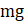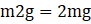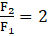Question 6. If the value of g suddenly becomes twice its value, it will become two times more difficult to pull a heavy object along the floor. Why?

Question 6.

If the value of g suddenly becomes twice its value, it will become two times more difficult to pull a heavy object along the floor. Why?

For lifting any body of mass ‘m’ the force(F1) required is equal to ‘mg’ where

g = acceleration due to gravity;

(Since Force= Mass × Accleration

Therefore F1 =Now if the value of ‘g’ suddenly becomes twice its value than force(F2) required will be

F2 =.

We can see thatTherefore it becomes difficult to pull a heavy object if value of ‘g’ becomes twice its value as double the force is required to overcome the acceleration produced by the force of attraction of earth acting in downward direction.